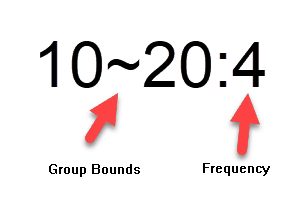﻿ Grouped Data

# Grouped Data

Top  Previous  Next

You can include grouped data information in data parameters using the following format:You can list these groups out in a data parameter, with the values separated by commas (or semicolons if you use a comma as a decimal point)

\$g=data(10~20:2,20~30:6,30~40:4,40~50:2)

This is equivalent to this grouped data table.

 Group f 10 < x <= 20 20 < x <= 30 30 < x <= 40 40 < x <= 50 2 6 4 2

All statistical commands (mean, median, standard deviation...) will utilize the group information when calculating their values.

You can access individual entries in the data parameter using references. For example, if you type \$f, the system will return  30~40:4.

You can access the group definition and frequency components of the table using the group and freq commands in the calculator.

{group(\$g)}                will return 30~40

{freq(\$g)}                will return 4